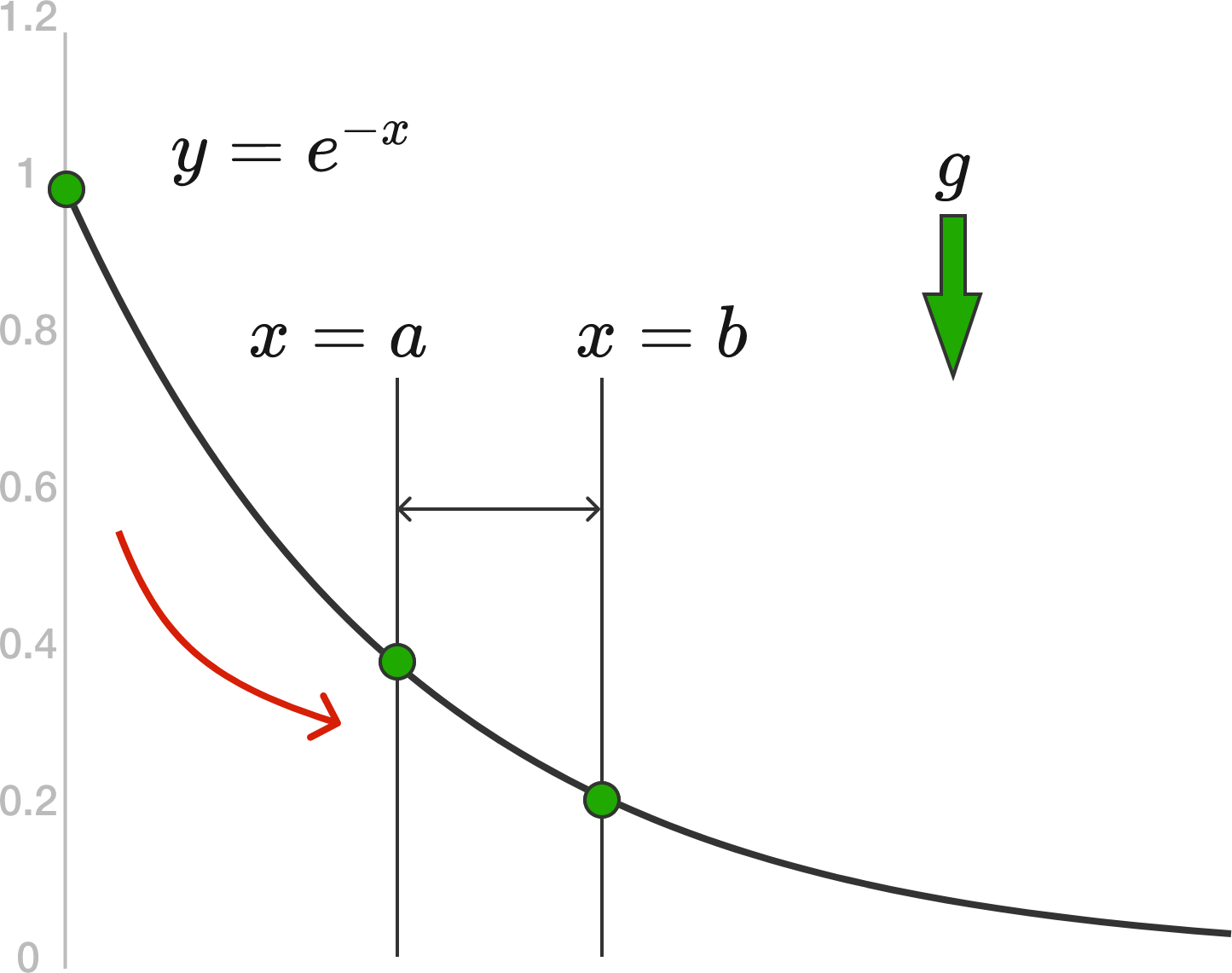# Going Down the Exponential SlideA bead slides under the pull of gravity, $g,$ down a frictionless wire segment in the shape of the curve $y = e^{-x}$, where $x$ is the horizontal direction and $y$ is the vertical direction. The bead starts from rest at $(x,y) = (0,1)$.

The time it takes for the particle to travel between $x=a$ and $x=b$ can be expressed as

$\large{t_{a,b} = \frac{1}{\sqrt{2g}} \int_a^b \sqrt{\frac{1 + P e^{Q x}}{1 + R e^{S x} }}\,dx},$

where $P,Q,R,$ and $S$ are integers.

Determine $P + Q + R + S.$

Note: The constant $e$ is Euler's number.

×